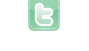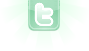## Greenfoot set more images

Hi, I got task. I need to change stop(red) semaphore to getready(orange and red) semaphore to ready(orange) semaphore and then to start(green) semaphore. And I don't know how. I only know function boolean but this one is only for two images and i need 4 images. Can someone help me please? PS. I need it quick thank u
off.nika wrote...
Hi, I got task. I need to change stop(red) semaphore to getready(orange and red) semaphore to ready(orange) semaphore and then to start(green) semaphore. And I don't know how. I only know function boolean but this one is only for two images and i need 4 images. Can someone help me please? PS. I need it quick thank u
Can i ask u how will code look? For example first image is red, second orange, third green and fourth greenandred ??? Thank you
because i have this. And I need to change images by clicking the mouse on the object(actor semaphore)
```import greenfoot.*;  // (World, Actor, GreenfootImage, Greenfoot and MouseInfo)

/**
* classic Semaphore
*
* @author Nicol Ševčíková
* @version 1.0
*/
public class Semaphore extends Actor
{
private int pressed;

public Semaphore() {
this.setImage("stuj.png");

}
public void act ()
{
this.setImage("priprav.png") ;

}
}
```
off.nika wrote...
Can i ask u how will code look? For example first image is red, second orange, third green and fourth greenandred ??? Thank you
```// global
private int timer;

// actions
timer++;
if (timer%60 = 1)
{
if (timer/30 == 2) set();
if (timer/30 == 3) start();
if (timer/30 == 4) getWorld().removeObject(this);
}```
This shows one way an int can be used in the way you want. A switch statement could be used in place of the multiple indented if statements. You could use this code as is, but you will need to define the methods that are called within the code.
off.nika wrote...
because i have this. And I need to change images by clicking the mouse on the object(actor semaphore) << Code Omitted >>
That's a bit different:
```// global
private int clickcount;

// in constructor

// actions
if (Greenfoot.mouseClicked(this))
{
clickcount++;
if (clickcount == 2) set();
if (clickcount == 3) start();
}
if (clickcount > 2 && ++clickcount == 30) getWorld().removeObject(this);```
Thank you. But shouldn't there be "getready.png" ?? Because its not showing pictures.
off.nika wrote...
Thank you. But shouldn't there be "getready.png" ?? Because its not showing pictures.
```private void getready()
{
}```
Or, you can replace line 5 in my last post with line 3 here.
i do this, but its still shows me errors. What do i have bad? sorry if i bother you Im new here ....
```   // global
private int timer;

private int pressed () {
timer++;
if (timer%60 = 1)
public void act ()
{

{
if (timer/30 == 0)
this.setImage("stuj.png");
if (timer/30 == 1)
this.setImage("priprav.png");
if (timer/30 == 2)
this.setImage("pozor.png");
if (timer/30 == 3)
this.setImage("start.png");
if (timer/30 == 4) getWorld().removeObject(this);
}
}
}
}```
off.nika wrote...
i do this, but its still shows me errors. What do i have bad? sorry if i bother you Im new here .... << Code Omitted >>
```private int count;

public Semaphore()
{
setImage("stuj.png");
}

public void act()
{
if (count < 3)
{
if (Greenfoot.mouseClicked(this))
{
count++;
if (count == 1) setImage("priprav.png");
if (count == 2) setImage("pozor.png");
if (count == 3) setImage("start.png");
}
}
else if (++count == 30) getWorld().removeObject(this);
}```
THank you so so so so much. I finally understood and it works. Thank you